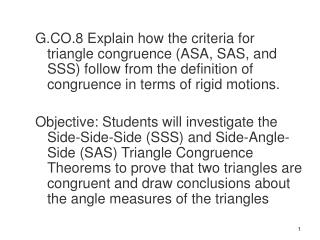DownloadDownload PresentationSide -Side-Side (SSS ) Congruent Postulate

# Side -Side-Side (SSS ) Congruent Postulate

Télécharger la présentation## Side -Side-Side (SSS ) Congruent Postulate

- - - - - - - - - - - - - - - - - - - - - - - - - - - E N D - - - - - - - - - - - - - - - - - - - - - - - - - - -
##### Presentation Transcript

1. G.CO.8 Explain how the criteria for triangle congruence (ASA, SAS, and SSS) follow from the definition of congruence in terms of rigid motions. Objective: Students will investigate the Side-Side-Side (SSS) and Side-Angle-Side (SAS) Triangle Congruence Theorems to prove that two triangles are congruent and draw conclusions about the angle measures of the triangles

2. Side-Side-Side (SSS) Congruent Postulate If 3 sides of one Triangle (Δ)are congruent ()to 3 sides of another triangle (Δ), then the triangles (Δs) are congruent ().

3. A Meaning: ___ ___ ___ If AB ED, AC EF & BC DF, then ΔABC ΔEDF. ___ B C ___ ___ E ___ ___ ___ ___ ___ ___ D F

4. Write the congruence statements for the two triangles then decide if the triangles are congruent.

5. Ex 2: Write the congruence statements then decide if the two triangles are congruent.SSS U Q 10 10 R S T

6. Ex 3: Write the congruence statements and then decide if the triangles are congruent SSS Y A X Z

7. You try (whiteboards)Write the congruence statements and then decide if the triangles are congruentSSS A Y X Z B C

8. You try (whiteboards)Write the congruence statements and then decide if the triangles are congruentSSS Y A X Z

9. Side-Angle-Side (SAS) If 2 sides and the included angle ()of one Δ are  to 2 sides and the included angle ()of another Δ, then the 2 Δs are .

10. Meaning: If BC YX, ACZX, and CX, then ΔABC  ΔZXY. Y B ) ( C A X Z

11. Ex 1:Write the congruence statements and decide if the triangles are congruent. SAS W Z X Y V

12. Write the congruent statements for the two triangles then decide if the triangles are congruent. Yes SAS

13. Example 3: Given: DE≅EB, CE ≅ EA (Mark the congruent sides) Decide if the triangles are congruent. YES SAS B A C E D

14. You try: Whiteboards Write the congruence statements and decide if the triangles are congruent.

15. theorem you would use

16. Write the congruence statements and decide if the triangles are congruent. SSS S Q R T

17. Write the congruence statements and decide if the triangles are congruent. SAS D A G R

18. Given: SD≅TC, CS≅DT Prove: ΔCST ≅ ΔDTS SSS ST =ST reflexive property D C K S T

19. Ex. 2: • Given: A is the midpoint of MT, A is the midpoint of SR. • Prove the triangles are congruent MA = AT def of midpoint SA = RA <A =<A Vertical angles

20. ASA QR = RS def of midpoint